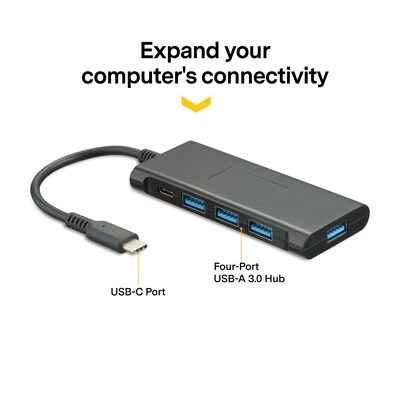Shared cart
Track an order
Reward not redeemed
You dont have enough points to redeem this reward.
Favorite list
Alternative Items

## Shop all NXT Technologies (160 products)

Filter Your Categories 160 items found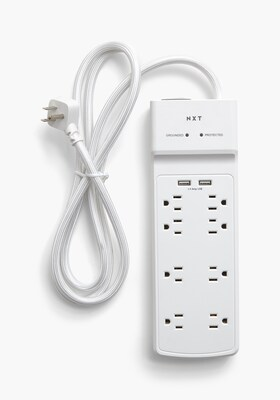\$28.99
Per each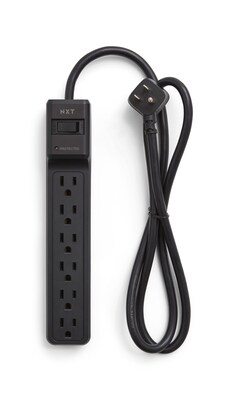\$10.79
Per each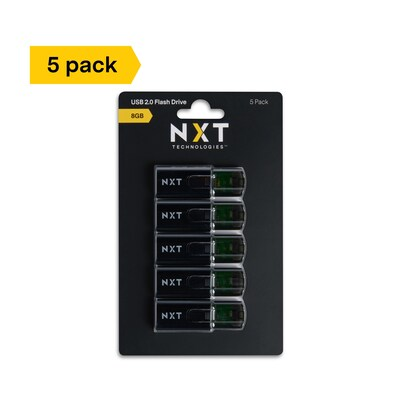\$29.99
Per pack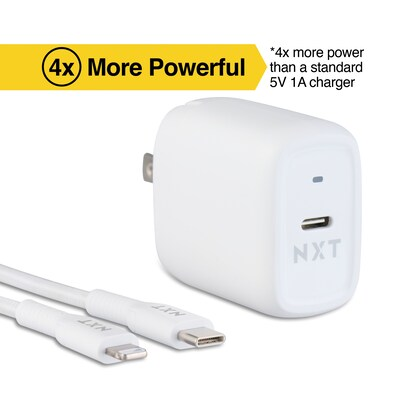\$33.99
Per each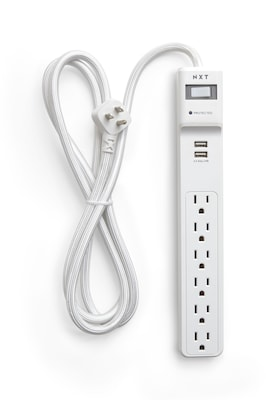\$23.79
Per each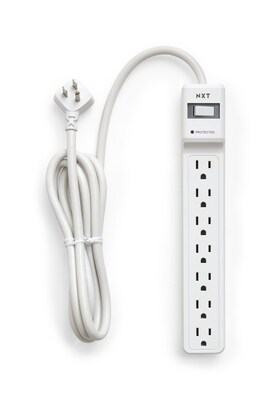\$23.59
Per each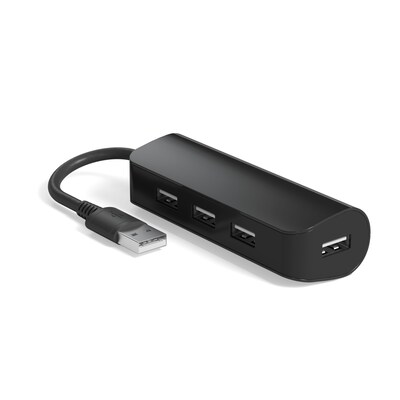\$15.59
Per each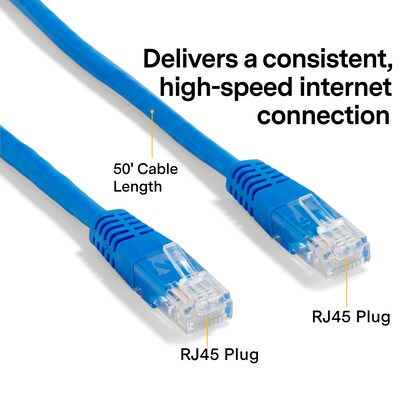\$14.59
Per each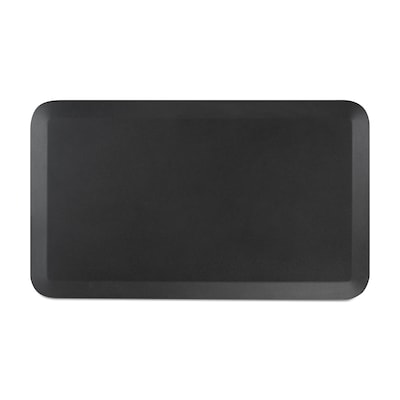\$53.99
Per each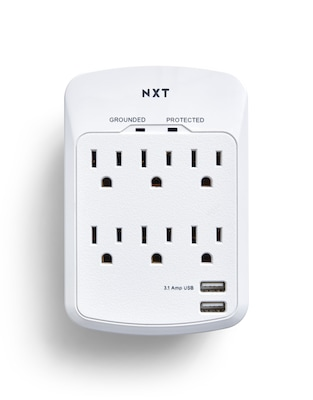\$19.99
Per each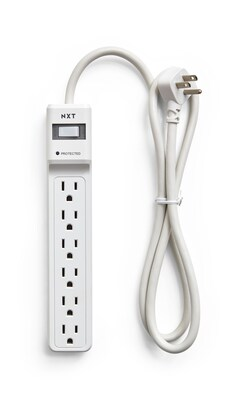\$10.79
Per each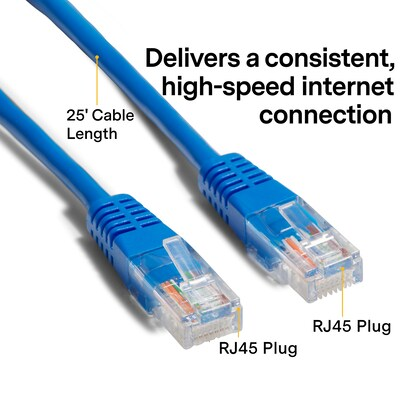\$28.99
Per each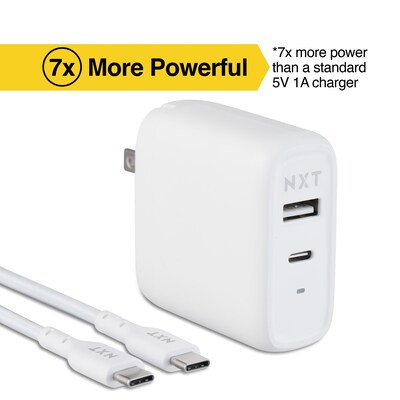\$29.99
Per each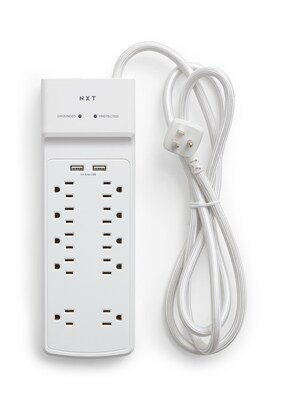\$34.99
Per each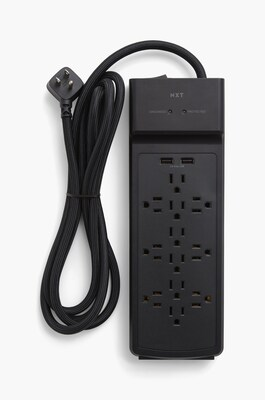\$40.99
Per each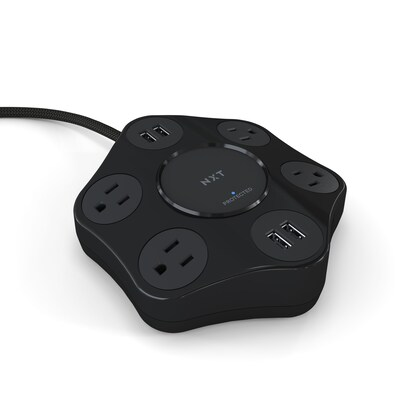\$35.99
Per each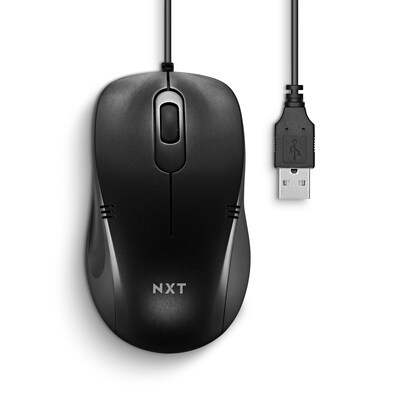\$8.99
Per each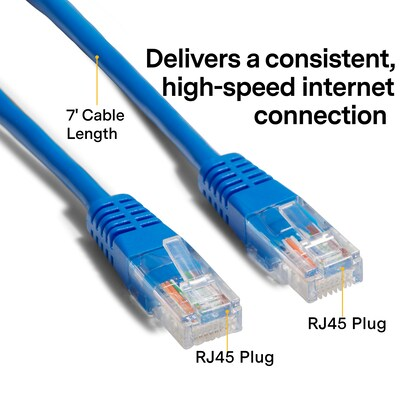\$4.79
Per each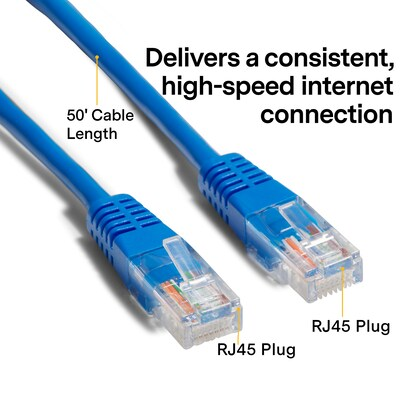\$37.99
Per each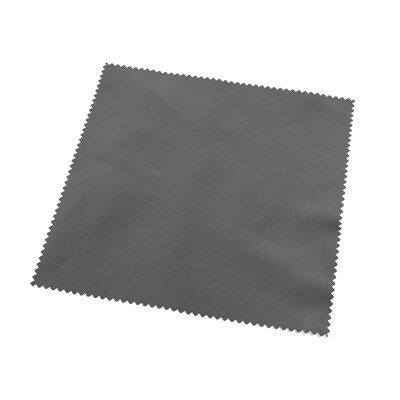\$5.49
Per each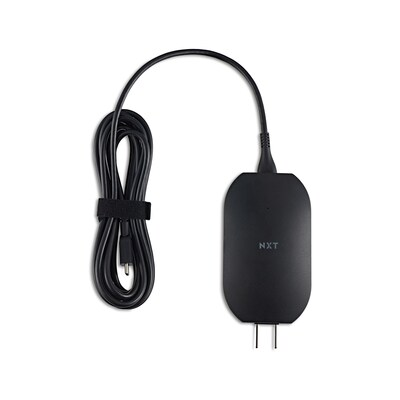\$49.99
Per each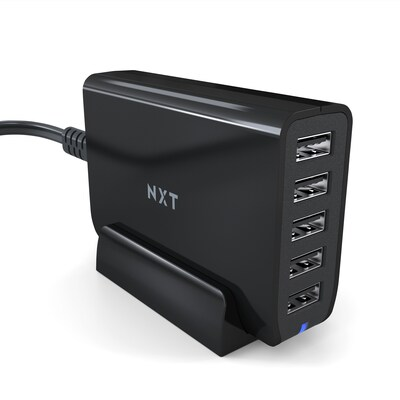\$29.99
Per each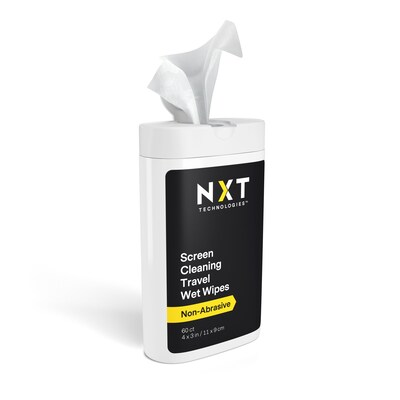\$5.49
Per each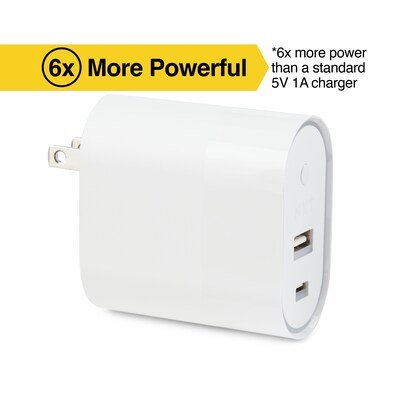\$24.99
Per each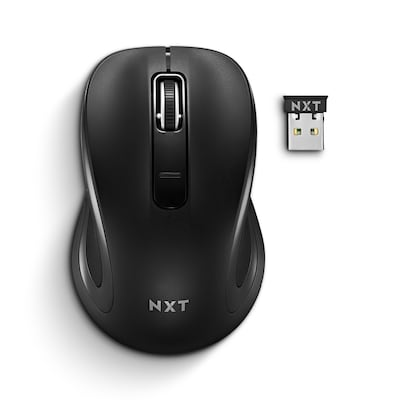\$16.99
Per each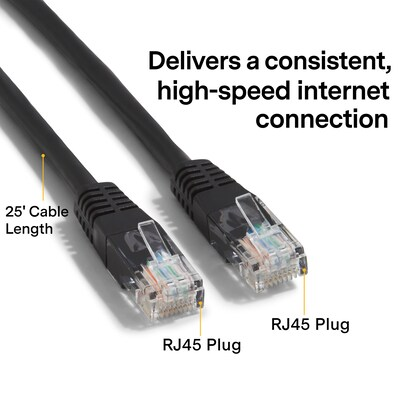\$34.99
Per each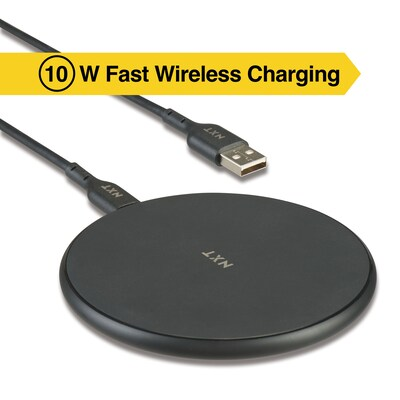\$28.99
Per each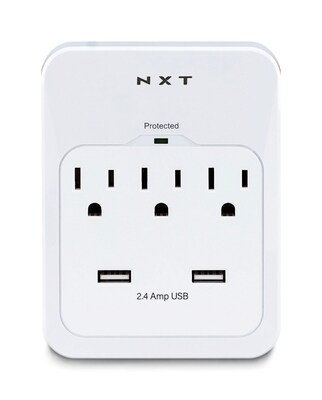\$19.59
Per each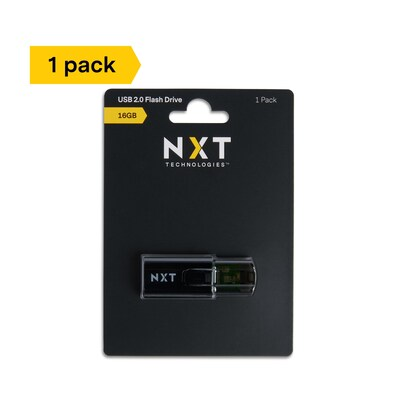\$8.99
Per each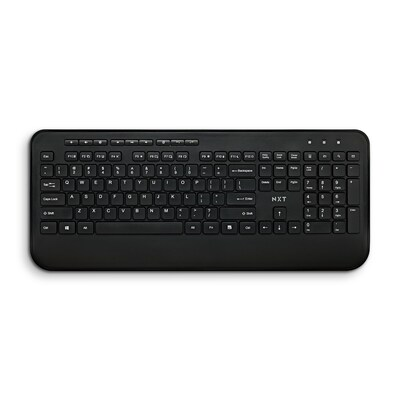\$29.99
Per each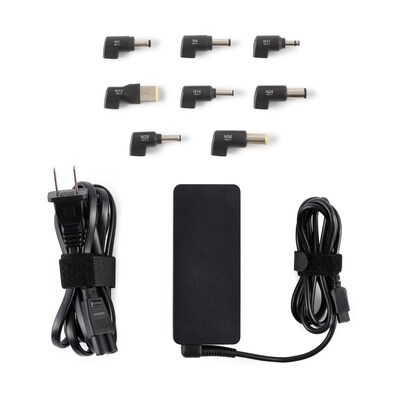\$58.99
Per each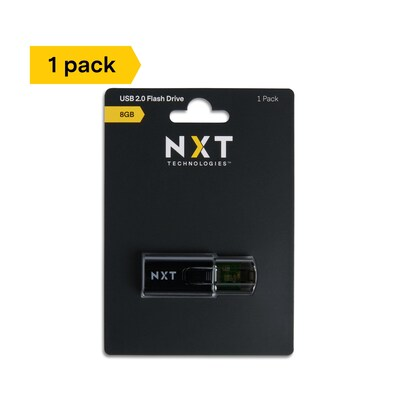\$7.99
Per each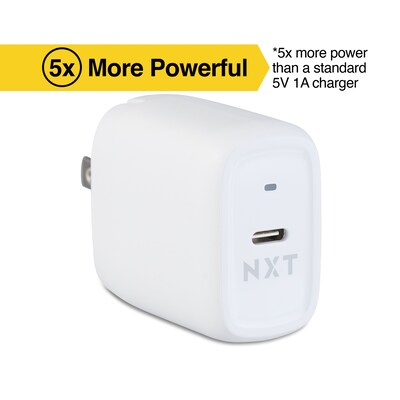\$18.99
Per each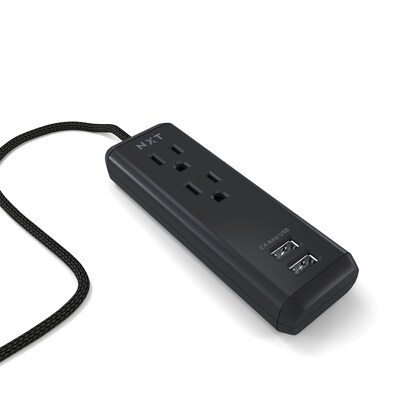\$15.59
Per each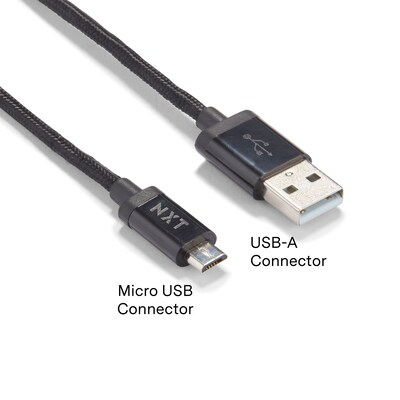\$11.99
Per each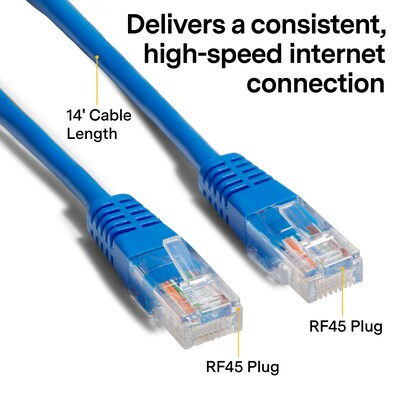\$12.99
Per each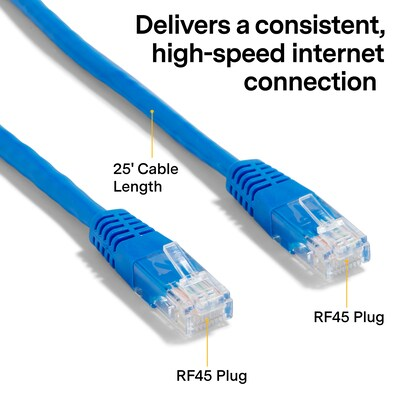\$20.59
Per each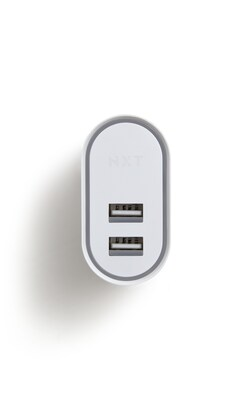\$25.99
Per each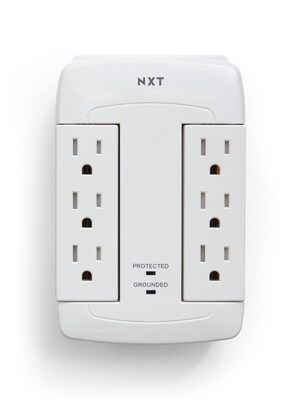\$17.99
Per each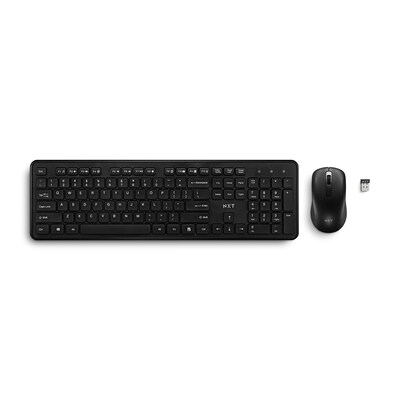\$24.99
Per each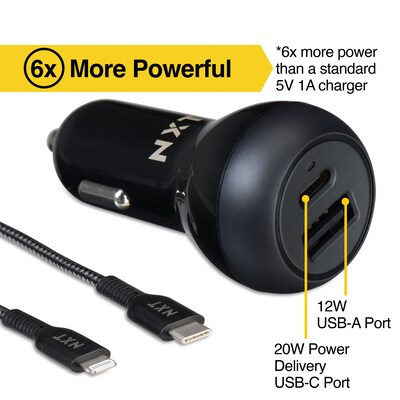\$29.99
Per each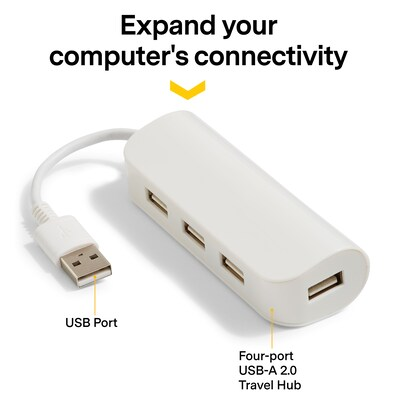\$13.99
Per each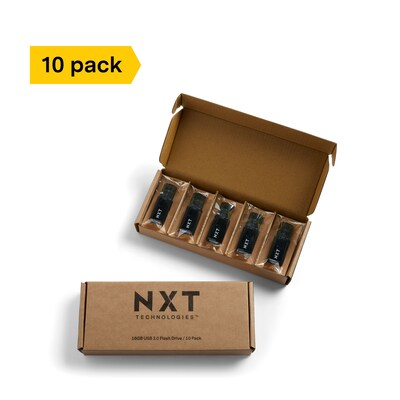\$69.99
Per pack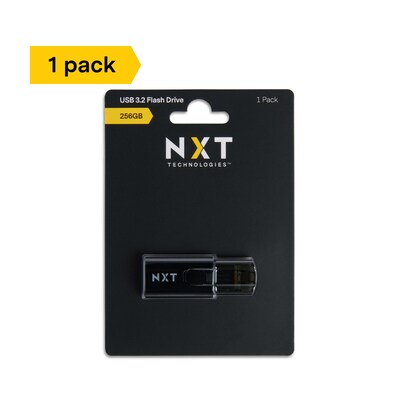\$59.99
Per each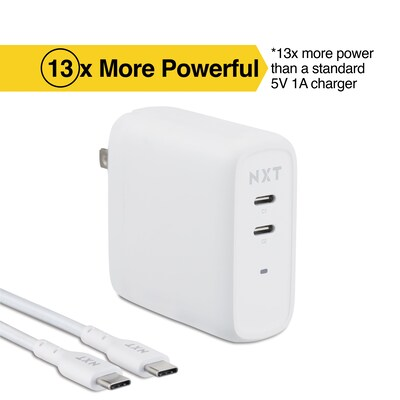\$55.99
Per each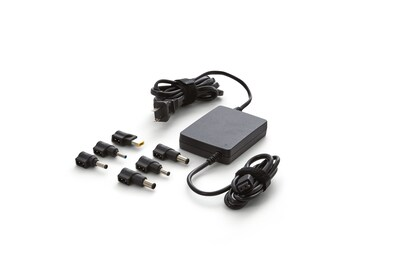\$70.99
Per each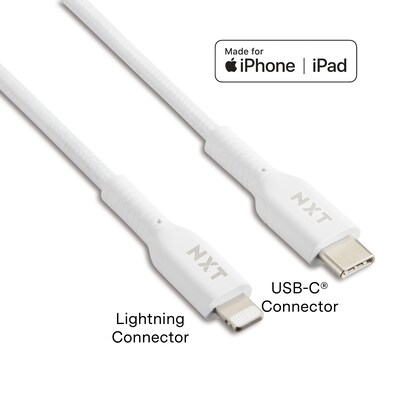\$17.99
Per each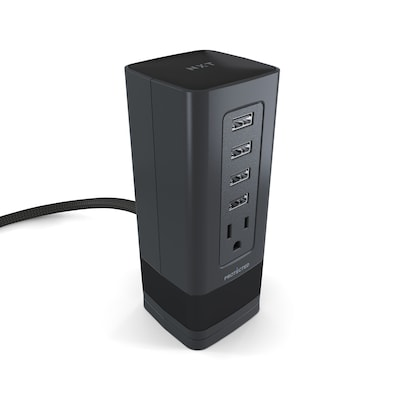\$29.99
Per each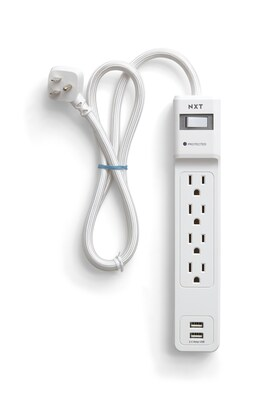\$17.99
Per each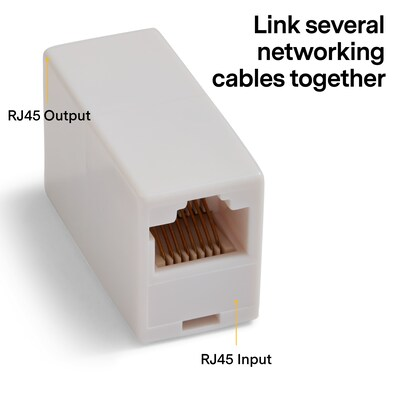\$14.59
Per each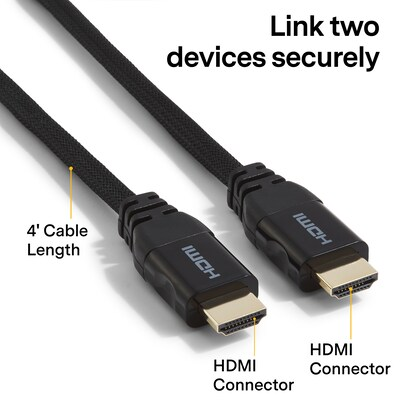\$14.99
Per each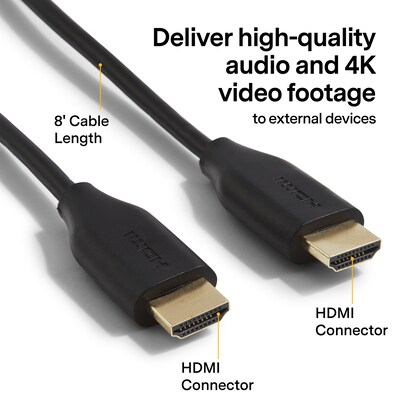\$35.99
Per each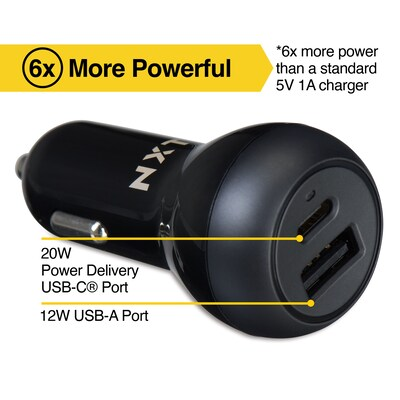\$24.99
Per each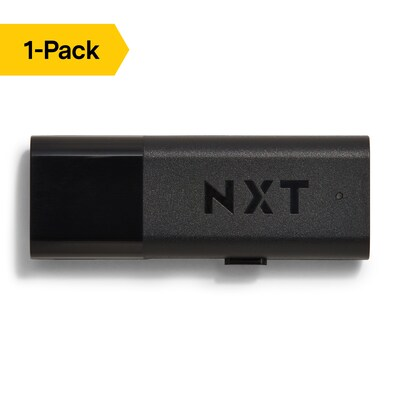\$11.59
Per each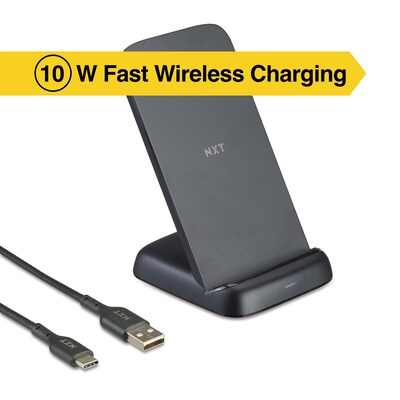\$33.99
Per each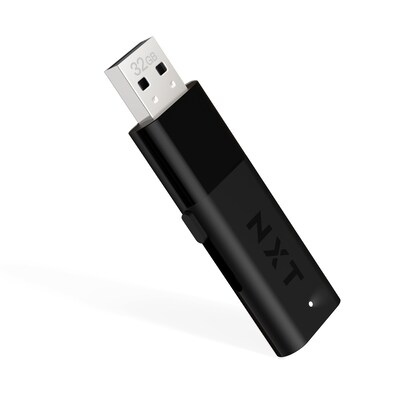\$12.79
Per each